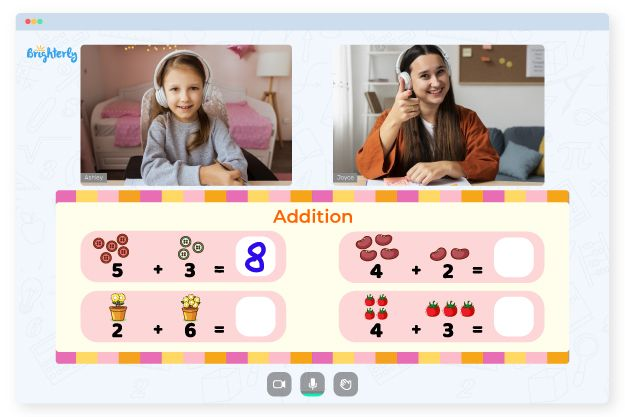# Kindergarten Addition And Subtraction Worksheets

Many kids are aware of the mathematical “take away” and “add” concepts by the time they join school. Most probably, they find it difficult to grasp the type of computation involved in subtraction and addition. An excellent way to change this situation is the use of free-to-download kindergarten addition and subtraction worksheets.

These tools typically incorporate colorful animated objects such as apples, school buses, pumpkins, and cars, which eases the process of teaching students about single-digit addition and subtraction worksheets. There are also mixed addition and subtraction worksheets kindergarten kids can use if they are more advanced learners.

## Benefits of Addition and Subtraction Worksheets

Addition and subtraction worksheets can include puzzles and color by number activities. All you have to do is download and print math worksheets for kindergarten addition and subtraction and start teaching your kindergartener these skills.

Math for Kids

Is Your Child Struggling With Math?
1:1 Online Math TutoringThe worksheets will help a child learn:

• Add up and subtract numbers.
• Match up numbers to corresponding images.

## Printable Addition and Subtraction Worksheets### Kindergarten Addition And Subtraction Worksheets PDF### Kindergarten Addition And Subtraction Worksheets PDF

Mixed Addition And Subtraction Worksheets Kindergarten### Kindergarten Addition And Subtraction Worksheets PDF

Addition And Subtraction Worksheets For Kindergarten### Kindergarten Addition And Subtraction Worksheets PDF

Math Worksheets For Kindergarten Addition And Subtraction

Adding and subtracting worksheets kindergarten are the tools kids usually enjoy. They learn about these two concepts in a fun and exciting way, while the images make it easier to follow and interpret information.• Is your child finding it hard to understand the concept of addition?
• An online tutor could be the answer.

Does your child struggle to grasp addition lessons? Try learning with an online tutor.